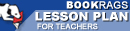Lesson Plans

# The Logic of Scientific Discovery Multiple Choice Test Questions

This set of Lesson Plans consists of approximately 102 pages of tests, essay questions, lessons, and other teaching materials.
 View a FREE sample## Part I: Chapter 1, A Survey of Some Fundamental Problems

1. What view does Popper take to analyze scientific thinking?
(a) Metaphysical.
(b) Epistemology.
(c) Philosophical.
(d) Linguistic.

2. What does Popper consider to contribute to the cosmology of empirical science?
(a) Inductive frameworks.
(b) Questions of fact.
(c) Metaphysical and philosophical ideas.
(d) Linguistic puzzles.

3. What is one approach to knowledge considered by Popper?
(a) Accidental.
(b) Common sense.
(c) Inductive.
(d) Purposive.

4. To what does Popper equate critical thinking?
(a) Common sense.
(b) Rational attitude.
(c) Deductive thinking.
(d) Inductive thinking.

5. What type of reasoning does Popper deny exists?
(a) Testable.
(b) Deductive.
(c) Scientific.
(d) Inductive.

6. What type of reasoning, according to Popper, is not rational?
(a) Deductive.
(b) Scientific.
(c) Inductive.
(d) Testable.

7. What type of reasoning does Popper believe inductive reasoning is?
(a) Metaphysical.
(b) Philosophical.
(c) Scientific.
(d) Rational.

8. What types of statements have no logical justification in which to draw conclusions?
(a) Logical.
(b) Inferential.
(c) Singular.
(d) Circular.

9. What does Popper suggest is the result of using logic to justify a statement?
(a) A loop that logic cannot escape.
(b) More problems.
(c) Theories.
(d) A solution.

 This section contains 3,601 words (approx. 13 pages at 300 words per page) View a FREE sample